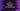# C++ proram to find the difference between two time periods using Structure## C++ proram to find the difference between two time periods using Structure:

In this post, we will learn how to find the difference between two user given time periods using structures. We will take the hour, minute and second values of both time periods and keep them in two variables defined by a structure. Then, we will find the difference between the two times and print the values.

### C++ program:

``````#include <iostream>
using namespace std;

struct Time
{
int hour;
int min;
int sec;
};

Time findTimeDiff(Time first, Time second)
{
Time diff;

if (second.sec > first.sec)
{
first.min--;
first.sec += 60;
}

if (second.min > first.min)
{
first.hour--;
first.min += 60;
}

diff.hour = first.hour - second.hour;
diff.min = first.min - second.min;
diff.sec = first.sec - second.sec;

return diff;
}

int main()
{
Time firstTime, secondTime, timeDiff;

cout << "Enter hour, minute and seconds for the first time : " << endl;
cin >> firstTime.hour >> firstTime.min >> firstTime.sec;

cout << "Enter hour, minute and seconds for the second time : " << endl;
cin >> secondTime.hour >> secondTime.min >> secondTime.sec;

timeDiff = findTimeDiff(firstTime, secondTime);

cout << "Difference between firstTime and secondTime is : " << endl;
cout << timeDiff.hour << ":" << timeDiff.min << ":" << timeDiff.sec << endl;
}``````

### Explanation:

• Time is a structure that can hold three int values hour, min and sec for hour, minute and seconds for a specific time.
• We have defined three Time variables firstTime, secondTime and thirdDiff to hold the first time, second time, and difference between first and second time.
• We are calling findTimeDiff to get the difference between firstTime and secondTime. This method returns the difference between firstTime and secondTime.
• In findTimeDiff, we are checking if the seconds value of second time is greater than the seconds value first time. If yes, we are decrementing the minute of first by 1 and incrementing the value of seconds of first by 60. Similarly, we are checking for the minute values.
• The difference of hour, minute and second are assigning to timeDiff variable. This method returns timeDiff.

### Sample Output:

This program will print the below output:

``````Enter hour, minute and seconds for the first time :
2 10 40
Enter hour, minute and seconds for the second time :
1 0 10
Difference between firstTime and secondTime is :
1:10:30``````

Here, we are finding the difference between 2 hours 10 minutes 40 seconds and 1 hour 0 minute 10 seconds. It prints the difference 1 hour 10 min 30 seconds.